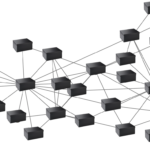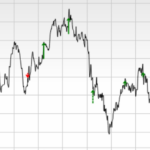# 5 types of loops you should avoidIn “Separation of compute, control and transfer” I talked about node-wise programming as a method we should embrace instead of trying to unroll the existing loops. In this article I get into loops and discuss a few types and how they can be run in a parallel form. Dependency is the big variable in each type: the lower the dependency on previous iterations, the better it can be parallelised. Another one is the known iteration-dimensions known before the loop is started.

The more you think about it, the more you find that a loop is not a loop.

# Loop types

The following loops are the ones I thought of. I did not find a collection of loop types, so they might be incomplete. You’ll find at many places that “some iterative problems let them better be described in while-loops, while others in for-loops”. Not the exact definition of the various iterative problems.

Notation-style is from Matlab/Octave.

## Independent, fixed dimension

This is code very easily run in parallel. This is the loop you want.

```[raw]for i=1:n
B[i]=A[i]*2;
end;[/raw]```

The only problem that needs to be solved is that each processor needs to do enough and memory is arranged well. These are the problems tackled in all GPU-programming guides. Any problem that can be described in this form, is a winner. For large enough n, this is the example of code that can be speed up easily.

Now to the loops you should avoid.

Each repetition the intermediate answer is updated, giving a result at the end. For instance the following loop.

```[raw]k=1;
for i=1:n
k=k*i;
end;[/raw]```

The result is the factorial of k and is not straight-forward to parallelize. One of the ways would be to split the loop in pieces and bring the results together at the end.

Some loops can be replaced by a single mathematical function (without any loops). That is without hardly any exception the fastest way to get the answer.

## Iterative search

Finding the answer by checking the intermediate-result each iteration. These are loops with intermediate answers, but without the fixed dimensions.

```[raw]i=0;
while result<10
i++;
result=A[i];
end;[/raw]```

If the programmer wanted to have the first element in A, which is smaller than 10, then the parallel implementation would be different if any element smaller than 10 had to be found. To speed things up, decisions are made in such code that we would have done differently assuming there are more cores in the processor. But say the first element needs to be found, striping-like techniques would best be used at the GPU such that each of 100 cores searches at element x+100. Thus, this type of loops can be very dependent on interpretation of the actual goal.

## Recursive

Recursive functions are not really loops, but I do want to mention them. Recursive functions are very close to human way of thinking in more complex problems. These loops are quite serial.

```[raw]function recur (a, b)
if a>0
recur (a-1, b*2+a)
end;
end;[/raw]```

These functions can be transformed to a simple function (Mathematical software are a great help here), or unrolled to stack-based loops (see below) or loops with intermediate answers. As a recursive function they cannot be run in parallel.

## Stack-based and backtracking

A stack-based loop needs more intermediate answers and keeps them at a stack. An example I like is to solve a maze: the moment a dead end is found, the current trace is marked dead and the loop continues a step back. Another one is parsing text.

A parallel function is made by finding split-points in the search-area such that each branch has the same weight. These loops (most times) don’t have fixed dimensions, so weighting the branches running-time can be tricky.

## Controlled

Within the loop there are control-statements (like “if…then”) or statements that alter the variables of the loop. Also while-loops are controlled code, as the result is checked each iteration. See also the iterative search.

```[raw]k=3;
for i=1:n
if mod(k,29)==0
k=3;
i=i-1;
else
k=(k-1)*k;
end;
B[i]=A[i]*k;
end;[/raw]```

Altering the loop while running is quite hard to interpret by compilers (both human and machine). So first, clean up the code by finding out how many times the code needs to be repeated. Second, decide if it is.

(Note that control-statements are expensive on GPUs (especially AMD’s) and should be avoided, if possible – in this case very doable as all possible values of k are known and very limited).

# Final words

To get multiples of 5, you can add 5 iteratively. It can also by done like in functional programming and multiply [1..20] with 5 to get multiples up to 100. In this case it is easy to spot, but not always. Also many programmers would still try to use old habits, making the following code a good idea but not efficient in the long run.

```[raw]rangerun i=0:0.001:pi, j=pi:0.001:2*pi
result[i,j]=sin(i)*cos(j);
end;[/raw]```

I strongly think the programmer should be made aware what he or she is actually doing: iterating, building up to an answer, or running a range of data-computations. The IDEs can support the developer in transforming non-performing loops into performing range-runs, while compilers can give hints and warnings when bad loops are used. Trusting the compiler will not make our old code magically perform as good as code with clean loops – that is a promise we have had since too long.

### Related Posts#### The Art of Benchmarking

...  where to startSeeking very advanced toolsFinding a few (types of) problematic areasActually finding all the problematic areas, but ...#### Birthday present! Free 1-day Online GPGPU crash course: CUDA / HIP / OpenCL

...  in GPU-development. This training mostly helps in avoiding some time-consuming pathsQ: I'm in Asia/America. Will you hold ...#### Problem solving tactic: making black boxes smaller

...  is the mother of all fuckups" on the wall, because we should be assuming we assume. Problem is that we want to have full control and ...#### Improving FinanceBench

...  Intel Xe. We have more interest in benchmarking different types of algorithms on all kinds of hardware than to compare ...